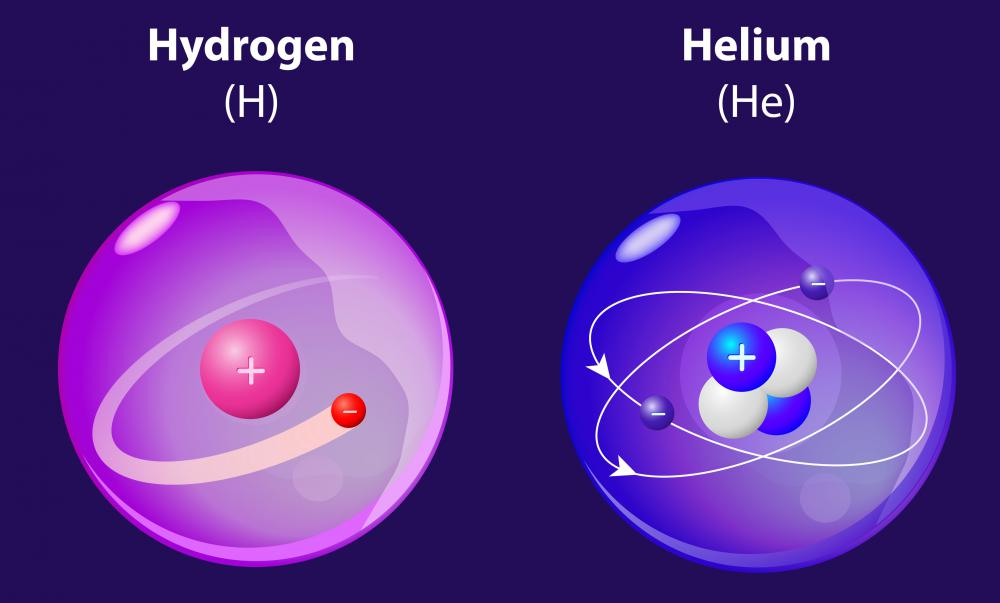# What Is the Atomic Radius?

Andrew Kirmayer
Andrew Kirmayer

The atomic radius is a size measurement for the atoms of a specific element. It indicates the distance between the nucleus of an atom and the outer edge of its electrons, or the distance between two atomic nuclei. An atom does not have a fixed structure, so its atomic radius is measured by dividing the distance between the nuclei of touching atoms in half. The radius can be different for the same atom depending one whether it is bonded or just next to another atom. Atomic size decreases further along each row of the periodic table when accounting for alkali metals to noble gases, and increases down columns.Atomic radius tables show the second-lightest element, helium, with the smallest radius, while the lightest element, hydrogen, is sixth from the bottom.

An atomic radius table is structurally different from the classic periodic table of elements. Helium has the smallest radius, while hydrogen, the lightest element, is sixth from the bottom for size measurement, and cesium is the largest atom. Neutral atoms range in size from 0.3 to 3 angstroms, and atoms and ions with one electron can be measured using the Bohr radius, determined by the orbit of the lowest energy electron in the atom.

The radius of covalently bonded atoms is different from that of touching atoms. Atoms that are bonded share electrons, and the radii of densely packed atoms, such as in a metallic structure, is different than if the atoms are just sitting next to one another. The van der Waals radius is used for atoms that are held together by weak attractions and not held together in a molecule. Adding electrons to an atom changes its atomic radius, so the ionic radius can vary depending on how many electrons orbit around an ion.

Atomic radius is based on the principle that atoms are spheres. This is not exactly the case, and the sphere model is only an approximate representation. The idea of spherical atoms helps to explain and predict how dense liquids and solids are, how atoms are arranged in crystals, and to calculate molecular shape and size. Atoms increase in radius down the rows of the periodic table, but increase in size dramatically between noble gases at the end of the row, or period, and the alkali metal beginning on the next row. This concept has been used in the development of quantum theory and is logical in relation to electron shell theory, which explains how many electrons can be in any particular orbit.

## You might also Like